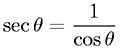Equations > Trigonometry > Trigonometric Properties > Reciprocal Property - Cosine and Secant

Reciprocal Property - Cosine and SecantLatex Code:

MathML Code:

 $\mathrm{sec}\theta =\frac1\mathrm{cos}\theta$

MathType 5.0: